# Introduction

During a long career,  V. Červený  contributed much to seismic ray theory. The initial impetus was for the determination of crustal structure from refraction profiles. Initial codes were for a 2-D model with corrections to represent 3-D propagation. One program was named seis81 which is encapsulated in the Computer Programs in Seismology (Herrmann, 2013)  program cseis96. The techniques are described n a number of publications (Červený et al, 1977; Červený, 1985; Červený, 2010;  Červený and Pšenčík. 2011).

cseis96 differs from the original seis81 in that the radiation patterns from point force and moment tensor sources was corrected and in   revised  output formats. The significant difference in making synthetics is that the formtion of the time series is make by the program cpulse96 after cseis96 is run.  The program cprep96 is a front end that simplifies the preparation input for seis81. However  cseis96 can run more complicated models if the input data set is created manually. The documentation for this is in PROGRAMS.330/VOLIX/DOC/seis81.txt.

As mentioned, seis81 was developed to interpret refraction line observations. The codes use asymptotic ray theory to make synthetics. The implication is that the codes cannot model phenomena such as the classical refraction or a Rayleigh wave. This tutorial investigates the use of these codes to make synthetics that can be used to model teleseismic P-wave receiver functions which assume a plane wave incident to the base of the structure.

In the process of testing this use, updates were made on November 1, 2022  to the source codes in PROGRAMS.330/VOLIX/src, specifically to cprep96, cseis96 and cpulse96. Changes were made in format statements since the testing required placing the source at a great depth to be able to approximate an incident plane wave. The numerical integration to define the ray path in cseis96 was upgraded to use double precision since there were significant problems in shooting a ray to a given receiver position when using very large source depths to approximate incident plane waves. In addition, changes were made to cpulse96 to permit a new way to define the time of the first sample and to restrict the rays contributing to the seismogram by specifying a range of acceptable ray parametes.

The ray tracing code works by shooting a set of rays through the structure until a pair is found that bracket the horizontal position of the receiver. At this point the angle of the ray leaving the source is refined so that the ray hits the receiver within a given accuracy. The ray so defined uses Snell's law locally at each interface. The final step is to account for the reflection and transmission at each interface and the geometrical spreading.

The program cpulse96 was initially written assuming that the source is to the left of the profile and waves propagate to the right in the +x direction. Thus the  RDD, RDS, RSS,. REX, RVF and RHF  Green's functions will be such that the motion of a P wave incident at the surface will be up on the vertical component and positive on the radial component or down and negative if the  initial motion is a dilation.  As will be show below, if the source is to the right of the array, then the horizontal  motion will be reversed.  Although the Green's fucntions have 'R' in their name, e.t., REX, the  motions should be interpreted as motions with respect to the direction of the horizontal x-axis rather as radial with respect to the source.  This means that computation of receiver functions for observations at distances less than the source position (e.g., in the negative x-direction), must have the R Green's function reversed, or if not, then the receiver function inverted.

For ease of making profiles, the Sac header variables STLA and EVLA will be undefined, e.g., -12345.0. STLO and EVLO will be the horizontal positions of the receiver and source. The distance DIST is defined at STLO - EVLO. Thus for sources to the right (more in +x direction) representing ways in the negative x-direction, the distance will be negative. Again this is for use in plotting. The parameter LCALDA= false int he Sac header so that the distances are nor recomputed from used int given latitudes and longitudes.

Given these header values, it is possible to use the gsac commands prs STLO or prs DIST to make record section profiles. in the examples below for the modeling of observations above a subduction zone, prs STLO is used.

Since this tutorial is directed toward modeling teleseismic P-wave receiver functions, only the EX Green's functions will be used since these only emit P waves at the source.

# Codes and scripts

The scripts for the examples below are contained in the file Cerveny.tgz. Download this file and unpack using the command

```
gunzip -c Cerveny.tgz | tar xf -
```

This will create a directory structure, such as
```Cerveny---
|--/Layer1-----
|              |--/DOIT
|              |--/DOPLT         |              |--/DOCLEANUP         |
|--/Layer------
|              |--/DOIT
|              |--/DOPLT          |              |--/DOCLEANUP
|
...
```
Note the names of the subdirectories. These will be the headings of each of the examples given below. Thus if an example has the heading Layerb, then the computational scripts will be in Cerveny/Layerb.

Since the objective of this tutorial is to model teleseismic P-wave receiver functions for observations above a subduction zone. There are some caveats.

First cseis96 is 2-D code. Thus a subduction zone is modeled as an  infinitely long 2-D structure and the source and receivers are on a line perpendicular to strike. If this were not true, then 3-D code would be required. The next limitation is that the receiver function analysis assumes a plane wave incident at the base of the structure while cseis96 uses a point source. If the point source is placed deep enough, then the incident wave field may be assumed to approximate a plane wave. However some experimentation is required to test this assumption.

The following sections consider a suite of models, ranging from a single layer over a halfspace model  to a complex subduction zone. For  the    plane layered halfspace models, the synthetics and receiver functions can be compared to more exact synthetics derived using the hspec96 and rftn96 codes.  The receiver functions computed  using hrftn96 can be compared to those obtained by running saciterd with the horizontal and vertical component synthetics derived from  cseis96.

The user has a choice when trying to model teleseismic waves incident from the right or the left of the structure.  One can fi the structure and make separate computations for with sources to the left and the right, or one can have the source to the left, but two models, were are reflections of the other.  Some experimentation in defining the source position for receiver function analysis, since one is interested in a certain set of ray parameters from the source (s/km) that reach the station. This will be illustrated in the examples.

in the examples below for the subduction model, the model extends down to about 300 km, but the source depth is 16000 km. Because of the different vertical extents, it is acceptable to ignore the upper structure to define the horizontal offset of the source in order for a given ray parameter to "hit" the receiver. A simple program ptoradian is provided to give an acceptable range of angles that cseis96 should use for efficient computations.

## cprep96

The program cprep96 prepares the data set for the cseis96 program by creating the file cseis96.dat. The format of that file is given in PROGRAMS.330/VOLIX/DOC/seis81.txt.  The command line flags are seen by the command cprep96 -h

` Usage: cprep96 -M model  [-DOP] [-DOSV] [-DOSH] [-DOALL] [-DOCONV] [-DOREFL]  [-DOTRAN] [-DEBUG] [-DENY deny ]  [-R reverb] [-N maxseg] [-HS sourcez] [-XS sourcex] -d dfile -M model  (default none )  Earth model file name -N nseg   (default 12   )  Maximum number of ray segments  -DOP      (default false)  Generate P ray description -DOSV     (default false)  Generate SV ray description -DOSH     (default false)  Generate SH ray description -DOALL    (default false)  Generate P, SV, SH ray description -DOCONV   (default false)  Permit P-SV conversions -DOREFL   (default false)  P-SV conversions on  reflection -DOTRAN   (default false)  P-SV conversions on  transmission -DENY     (default none )  file with layer conversion denial -R reverb (default none )  file with maximum number of multiples in layer -HS sourcez(default 0.0  )  source depth  -XS sourcex(default 0.0  )  source x-coordinate  -d dfile  (default none )  distance file     dfile contains one of more lines with following entries        DIST(km) DT(sec) NPTS T0(sec) VRED(km/s)            first time point is T0 + DIST/VRED            VRED=0 means infinite velocity though -?        (default none )  this help message  -h        (default none )  this help message `

The importance of the -XS sourcex command line flag is illustrated in Figure 1. This figure shows the ideal ray paths to each receiver associated with an incident plane wave. Since cseis96 can only use point sources, it is assumed that the point source (red circle) is at a depth such that the wavefront is effectively planar.  To preserve the ray geometry, the source must be moved horizontally as the receiver position changes.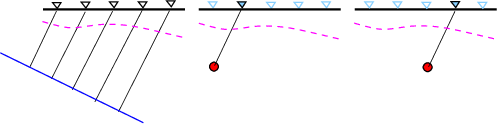Fig. 1. Sketch showing receiver and effective source positions. Left) plane wave incident on 2-D structure.
Center and right) point source locations to preserve the angle of incidence at the receiver.

The -R reverb is useful to reduce the number of rays considered. The format is simple, a list of layer number - bounce pairs, e.g.,

`1 32 33 34 1`

which means, for example that at most 3 ray segments are permitted in layer 2. For P-SV waves this would permit
8 rays, e.g., PPP, PPS, PSP, PSS, SSS, SSP, SPS and SPP.   If this option is not ste the cprep96 may generate a lot of rays, perhaps 40,000 for a 4 layer model.

The format for cseis96.dat  is given in the aforementioned seis81.txt. Just before the ray description there is a line created by cprep96 which reads

`    1.0000    3.1416   -0.0628   -3.1416   -3.1416    0.0628    3.1416    0.0010`

The documentation for this line is

`     12) One card, quantities that control the basic system of initialc         angles in the two-point ray tracing,etc.c         dt,amin1,astep1,amax1,amin2,astep2,amax2,ac    format(8f10.5)c           dt...     time step in the integration of the ray-tracingc                     system. dt should be greater than zero.c                     If dt.lt.0.00001, then dt=1.c           amin1,astep1,amax1... determine the system of initialc                     angles for primary reflected waves (and possiblyc                     for other manually generated elementary waves,thec                     first element of which strikes the interface situ-c                     ated below the source)c           amin2,astep2,amax2... determine the system of initial anglesc                     for the direct waves (and possibly for other manuallyc                     generated elementary waves,the first element ofc                     which strikes the interface above the source).c           ac...     required accuracy of integration of the ray tracingc                     system. Recomended values: 0.0001 - 0.001.`

You may wonder why the limits in the example line are π to -π and -π to π. The code permits velocity gradients. Thus if velocity increases with depth and one wanst a ray from the source to a receiver above the source, the ray will go upward  at short distances  and downward for large horizontal distances. The range given here covers all possibilities. However examining all possibilities is time consuming. For the purpose of modeling teleseismic P waves, this line will be changed to make the computations more efficient

Following this is the ray description for each ray

`    0    2    2    1    0    2   -2    1    0    2    2   -1    0    2   -2   -1`
which represent the rays P2P1, S2P1, P2S1 and S2S1, respectively. In this notation the 0 indicates that an acceptable ray can go up or down from the source, the initial 2 says that there are two ray segments, which is followed by a layer number and whether the ray of P (positive) or S (negative).

Note again that the number of rays can quickly become large if conversions between P and SV are permitted.  For example if there is a single layer over a halfspace, then there will be 2 rays in the halfspace, 4 direct rays reaching the surface, as in the example above. If the first set of multiples are permitted in the layer, then there will be 16 rays, e.g., P2S1S1P . This is easily understood if one writes the ray type for each segment in binary, using 0 for P and 1 for S. Thus one would have 0 or 1  for the halfspace, and then 0 or 1 for each segment in the layer. Thus this example may be written as 0 ro the halfspace and 110 for the layer.  If there are many layers, it will be possible to have 10's of thousands of rays/.

## cseis96

The next program that is executed is cseis96. Its command lines are obtained by executing cseis96 -h which gives

` Usage cseis96 [-v] [-P] [-?] [-h]  -v    (default false) verbose output  -R    (default false) generate file for CRAY96  -?    (default false) this help screen  -h    (default false) this help screen`

The output of this program consists of the file cseis96.amp which is used by cpulse96 and cseis96.trc if cseis96 -R  flag is run.  The cseis96.try is used by cray96.

## cray96

The program cray96 plots the structure and the individual rays, with colors indicating P or S segments.  This requires the use of the -R flag with  cseis96. As will be seen below, such a plot can be very complicated, and it is recommended that only one receiver position be considered. This used the output contained in the file cseis96.trc, whh can be very largeThe command line defines the boundaries for the plot.

` Usage: cray96 -XMIN xmin -XMAX xmax -ZMIN zmin -ZMAX zmax -v   -XMIN [xmin]    (default 0)       : Minimum X for plot  -XMAX [xmax]    (default=400.0)   : Maximum X for plot  -ZMIN [zmin]    (default=0.0)     : Minimum Z for plot  -ZMAX [zmax]    (default = 50.0)  : Maximum Z for plot  -v      (default = false) : verbose output  -?      (default = false) : This help screen  -h      (default = false) : This help screen`
This program is very informative if there is only one distance plotted. If there are many distances, then one will get  a idea of the range of ray paths that reach the stations.

## cpulse96

Finally the program cpulse96 uses the cseis96.amp file created by cseis96 to make the synthetics.  The command line options of this program are seen by executing the command
cpulse96 -h. The output of this program the in the ASCII file96 format which can be converted to Sac files using f96tosac -B.

` USAGE: cpulse96 [ -v  ] [ -t -o -p -i ] -a alpha -l L [ -D -V -A]  [-F rfile ] [ -m mult]  [ -OD -OV -OA ] [-Z]         [-Q] [-DELAY delay [-EQEX -EXF -ALL] [ -PMIN pmin -PMAX pmax ]  [-?] [-h]  -v          Verbose output  -t          Triangular pulse of base 2 L dt  -p          Parabolic Pulse of base  4 L dt  -l L        (default 1 )duration control parameter  -D          Output is ground displacment  -V          Output is ground velocity (default)  -A          Output is ground acceleration  -F rfile    User supplied pulse  -m mult     Multiplier (default 1.0)  -OD         Output is ground displacement  -OV         Output is ground velocity  -OA         Output is ground acceleration  -Q          (default false) do causal Q  -DELAY delay (default use t=t0+x/vred for first sample,              else use delay seconds before the first arrival  -EXF        (default) Explosion/point force green functions  -EQEX       Earthquake and double couple green functions  -ALL        Earthquake, Explosion and Point Force   -Z          (default false) zero phase triangular/parabolic pulse  -PMIN pmin -PMAX pmax              If pmin and pmax have the same sign, then rays               with ray parameter |pmin|<= p <=|pmax| are  used.              A positive sign means a ray from source               propagates in the  +x direction, and a negative              in the -x direction  -?          Write this help message  -h          Write this help message`

As mentioned above,  the trace header fields have LCALDA=false, STLA=-12345, STLO=-12345, STLO= station x-coordinate, EVLO= station x-coordinate. Thus one can plot a record section of receiver positions in gsac using the command prs stlo .

The code update of November 1, 2022 added the -DELAY delay and -PMIN pmin -PMAX  pmax command line flags.  The reason for the  -DELAY delay  is as follows. The specification of desired horizontal distances in the dfile  used by cprep96 consists of lines containing the following

`DIST(km) DT(sec) NPTS T0(sec) VRED(km/s)            first time point is T0 + DIST/VRED`
an example of which is
` 00.0     0.125   1024   -10.0 6.0 10.0     0.125   1024   -10.0 6.0 20.0     0.125   1024   -10.0 6.0`
For the emulation of the teleseismic arrival, which will be approximated by placing the point source at a large depth, one would have to manually change the TO so some large value and have VRED= 0.0.  The -DELAY delay option says to start the synthetics delay  seconds before the first arrival.

The resulting output of cseis96 is; the file cseis96.amp which looks like the following.
```model.d 10   0.00000 200.00000   0.00000  10.00000                        Source is at (0,200)  0.1000E+02  0.1250E+00      1024 -0.1000E+01  0.6000E+01   	Distance 1  0.2000E+02  0.1250E+00      1024 -0.1000E+01  0.6000E+01	Distance 2
0.3000E+02  0.1250E+00      1024 -0.1000E+01  0.6000E+01  0.4000E+02  0.1250E+00      1024 -0.1000E+01  0.6000E+01  0.5000E+02  0.1250E+00      1024 -0.1000E+01  0.6000E+01  0.6000E+02  0.1250E+00      1024 -0.1000E+01  0.6000E+01  0.7000E+02  0.1250E+00      1024 -0.1000E+01  0.6000E+01  0.8000E+02  0.1250E+00      1024 -0.1000E+01  0.6000E+01  0.9000E+02  0.1250E+00      1024 -0.1000E+01  0.6000E+01  0.1000E+03  0.1250E+00      1024 -0.1000E+01  0.6000E+01    1   10 0.3727E+02 0.4561E-02 0.7823E-02 0.3142E+01 0.0000E+00-0.1107E+01    1 0.2800E+01 0.6000E+01 0.0000E+00 0.2800E+01 0.6000E+01    1    9 0.3655E+02 0.4284E-02 0.8160E-02 0.3142E+01 0.0000E+00-0.1148E+01    1 0.2800E+01 0.6000E+01 0.0000E+00 0.2800E+01 0.6000E+01    1    8 0.3590E+02 0.3963E-02 0.8487E-02 0.3142E+01 0.0000E+00-0.1190E+01    1 0.2800E+01 0.6000E+01 0.0000E+00 0.2800E+01 0.6000E+01The output here are 	ray number             - ray one	distance number        - 10 means here means the 10'th distance entry, which is  100 km here	horizontal amplitude   - these are used to make the synthetic	vertical amplitude	horizontal phase	vertical phase	pnew                   - For the first entry this is -1.107 radians, or and angle of 63.44 degrees measured upward                                 from the horizontal. This is equivalent to an incident angle of 90 - 64.44 = 25.56 degrees (true is 26.56)                                 There is a rounding error in the presentation of -0.1107E+01.
mwave                    1 means ray leaves the source as P	ros                      Source density is 2.8 gm/cm3	vels                     Velocity of ray type, e.g., P, at source is 6.00 km/s	sumtq	rsrf                     Density at receiver 2.8 gm/cm3
vsrf                     Velocity at receiver to this ray is 6.00 km/s```
This output does not have any information about the ray identified by the ray number. That information is contained in the file cseis96.dat,.

Lets consider a receiver function for the observation at a distance of 100 km. Since this is a halfspace, the angle of incidence at the surface is atan ( 100/200) = 26.56 degrees and the ray parameter is p = sin(26.56)/6.0 = 0.0745 s/km. The seismograms to be used are 0010002000.ZEX and 0010002000.REX. Now run saciterd

`saciterd -FN 0010002000.REX -FD 0010002000.ZEX -RAYP 0.0745 -D 10 -ALP 1.0`
which creates the Sac file decon.out. Also compute the theoretical using hrftn96 with the command
`hrftn96 -M model.d -NSAMP 1024 -DT 0.125 -ALP 1.0 -P -RAYP 0.0745 -D 10`

which creates the Sac file hrftn96.sac.

# Test Cases

The following test cases were designed to learn how to adapt the code for the purpose of modeling teleseismic P-wave receiver functions. The sequence of tests
leading to the images shown in this tutorial can be obtained  by executing the following commands in order:

`(cd Layer1; DOIT;DOPLT;DOCLEANUP)(cd Layer2;DOIT; DOPLT;DOCLEANUP)(cd Layer3;DOIT; DOPLT;DOCLEANUP)(cd NsubductionReverbF/;DOIT;DOPLT;DOSACITERD;DOREC)(cd NsubductionReverbR/;DOIT;DOPLT;DOSACITERD;DOREC)(cd DOCMPRFTN;DOCMP)`

## Layer1

This discusses running the code and also addresses the ability of this ray tracing code to approximate incident plane waves.
Everything is in Layer1

## Layer2

Here the ability to filter rays by ray parameter is done by modifying the cseis96.dat file.
Everything is in Layer2

Compared to the Layer3 example, these computations are faster since only a few rays are computed. In the Layer3 example, all rays are computed using cseis96 and the results are filtered.

## Layer3

Here the ability to filter rays by ray parameter is done by the command line of cpulse96.
Everything is in Layer3

## Initial subduction model

The initial test model is based on the image image from https://www.chegg.com/homework-help/questions-and-answers/figure-56-generalized-east-west-profile-center-nazca-plate-eastern-side-andes-mountains-ho-q52257549

. The model used here is shown in the next figure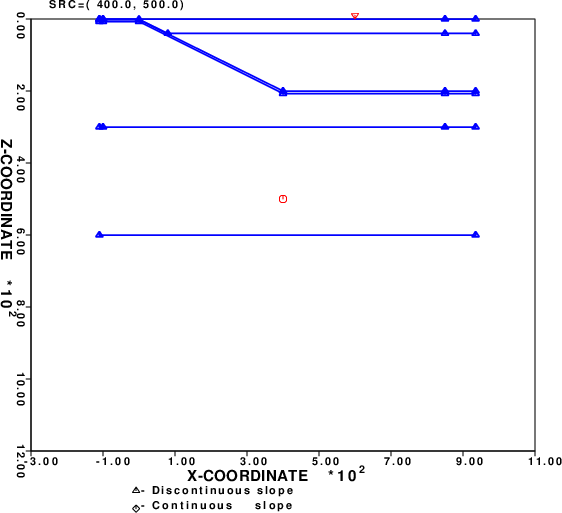The P velocities in the layers are 6.0, 8.0, 6.0, 8.0 and 8.5 km/s from top to bottom. The layer boundaries on the right side of the model are at 0, 40, 200, 207 and 300 km. (The lower boundary at about 600 km is inserted to that the source is oin a layer).

The layer boundaries use a simple format that is described in the tutorial at PROGRAMS.330/DOC/OVERVIEW.pdf/cps330o.pdf. Thus the layering consists of linear segments.

NOTE: This is not a restriction of cseis96. The layering can be specified to be smoother by uaing cubic splines - see the documantation at PROGRAMS.330/VOLIX/DOC/seis81.txt.

To create synthetics to emulate teleseismic arrivals and for computational efficiency, the processing scripts will select a target x-coordinate, xrec, for the station. It will then be neceasary to specifiy the source location, e.g., (xsrc, zsrc) where the zsrc is much larger than the structure thickness. In this case a  simple approximation is that the horizontal offset from the source will be xoffset =zsrc tan ( p V) where  p is the ray parameter of  the teleseismic arrival and V is the P-velocity at the source depth. If the ray is propagating in the +x direction, then xsrc = xrec - xoffset. If  the ray is propagating in the -x direction, e.g., a reverse profile, then xsrc = xrec + xoffset.

To facilitate the specification of the range of acceptable angles for the Cerveny code, a simple Fortran program was written. If the source depth or velocity change, just modify in the definitions at the beginning of the code. The code, ptoradian.f , compilation and output are as follow:

```      program ptoradian
c-----
c     This program considers at range of teleseismic
c     ray parameters typically observed. It then
c     converts the ray parameters to angles in radians
c     for use with the Cerveny code. In addiiton
c     it gives the horizontal offset for a given source
c     depth.
c
c     It is assumed tha tht source depth is much greater than
c     the thickness of the structure being modeled.
c-----
c     define the velocity of the P wave at
c     the source depth
c-----
vel=8.5
c-----
c     define the source depth deemed sufficient for a plane-wave
c     approximation
c-----
depth = 16000.0
call bdoit(0.045,0.055,vel,depth,.true.)
call bdoit(0.055,0.065,vel,depth,.false.)
call bdoit(0.065,0.075,vel,depth,.false.)
end

real plow, phgh, vel, depth
real anglow, anghgh, angmid, thetamid
real xoff
real pi2

write(6,1)depth,vel
endif
1 format('    Mapping of ray parameter range to horizontal offset ',
2'    for source depth of',f10.2,' and velocity of ',f10.3,' km/s'/
3'  p range (s/km)  Theta   Xoffset            Forward Rays (+x) ',
4'             Reverse Rays (-x)')

pi2 = 3.1415927/2.0
call getang(plow,vel,anglow)
call getang(phgh,vel,anghgh)
angmid = 0.5*(anghgh + anglow)
thetamid = angmid * 180.0 / 3.1415927
c-----
c     the horizontal offset is x=depth*tan(angmid)
c-----
xoff = depth*tan(angmid)
c-----
c     get the angles in radians for the forward and reverse
c     profiles
c     The Cerveny code measures angles with respect to the
c     horizontal. Upward rays are negative.
c     Rays in the +x direction, the forward direction, will
c     have angles in the range [0, -pi/2].
c     Rays in the -x direction, the reverse direction, will
c     have angles oin the range [-pi/2, -pi]
c-----
flow = -pi2 + anghgh
fhgh = -pi2 + anglow
rlow = -pi2 - anglow
rhgh = -pi2 - anghgh
write(6,2)plow,phgh,thetamid,xoff,flow,fhgh,rlow,rhgh
2 format('[',f6.3,' to',f6.3,']',f7.3,f10.3,5x,
1      2('[',f10.4,'  to',f10.4,']',5x))
return
end

subroutine getang(p,vel,ang)
real p, vel, ang
real pi2
pi2 = 3.1415927/2.0
ang = asin(p*vel)
return
end

```

```gfortran ptoradian.f
a.out

Mapping of ray parameter range to horizontal offset and ray angles in radians
for source depth of  16000.00 and velocity of      8.500 km/s
p range (s/km)  Theta   Xoffset            Forward Rays (+x)              Reverse Rays (-x)
[ 0.045 to 0.055] 25.180  7522.338     [   -1.0843  to   -1.1783]     [   -1.9633  to   -2.0573]
[ 0.055 to 0.065] 30.705  9502.150     [   -0.9854  to   -1.0843]     [   -2.0573  to   -2.1562]
[ 0.065 to 0.075] 36.572 11870.596     [   -0.8795  to   -0.9854]     [   -2.1562  to   -2.2620]

```

In the examples that follow, the 0.055-0.065 range of ray parameter is considered.

By default cprep96 will compute all ray conversions, which will create a huge number of rays. The -R reverb option to cprep96 is used to restrict the number of boundings in the layers of the model to 3,3,1 and 1, respectively. the 3 means that ion a given leyer, PPP, PPS, PSP, PSS, SSS, SSP, SPP and SPP are considered, which should be sufficient for this study.

The following tutorials consider wave propagation with receiver positions from 50 to 8000 km along the surface of the model above. At 800 km, for the the forward model, the way interaction should approximate what would be expected for a single layer over a halfspace, which can be compared to the output of hrftn96 to test the code. This is why cseis96 computations were extended to double precision when computing the ray from the soruce to the receiver.

### Forward profile

The synthetics for a forward profile are computed. In addition saciterd is created to make a p-wave receiver function profile as a function of the station longitude. Everything is in Forward profile

### Reversed profile

The synthetics for a forward profile are computed. In addition saciterd is created to make a p-wave receiver function profile as a function of the Everything is in Reversed profile

### Discussion

As expected the synthetics and receiver function show the effect of the 2-D structure. It us useful to compare the RFTN plots side-by-side:
 Forward Profile (+x)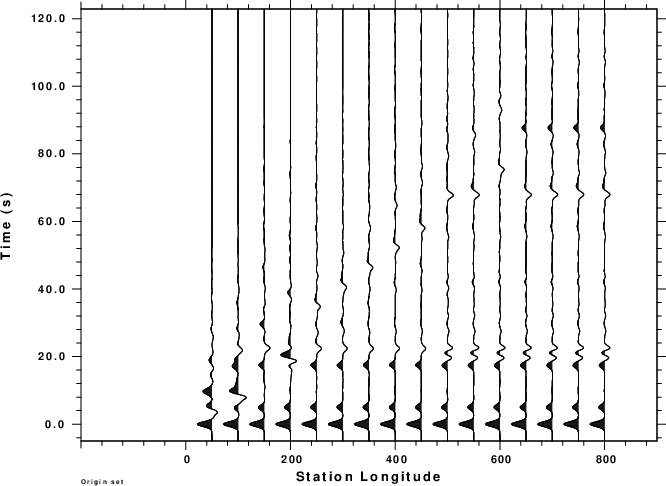Reversed Profile (-x)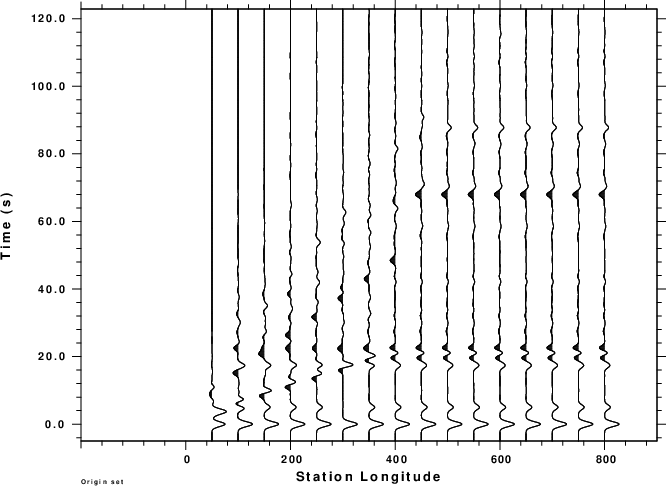First note that the RFTN's for the reverse profile are reversed in sign. This is because the REX Green's function does not show radial displacements, but displacements that are positive in the (-x) direction. This plot could be flipped by multiplying the traces by -1!

The traces look different in the 0 - 200 km range for the station position. For this reversed profile one sees the arrivals trapped within the wedge. The only disturbing feature is the shape of the receiver function at about 20 sec after the first "bump". To understand the difference, the following figure compares the receiver functions generated by hrftn96 for the simple single-layer over a halfspace (Simple.sac) and for a five layer flat model (Layered.sac) corresponding to the flat subduction structure at the right of the model. Finally the receiver functions corresponding to the 2-D forward model at receiver position 600 km are given in Forward600.sac while Reverse600.sac is for the reversed profile. In performing this comparison the reverse profile receiver function were multiplied by (-1) for comparison, thus converting modtion in the (-x) direction to radial with respect to the source.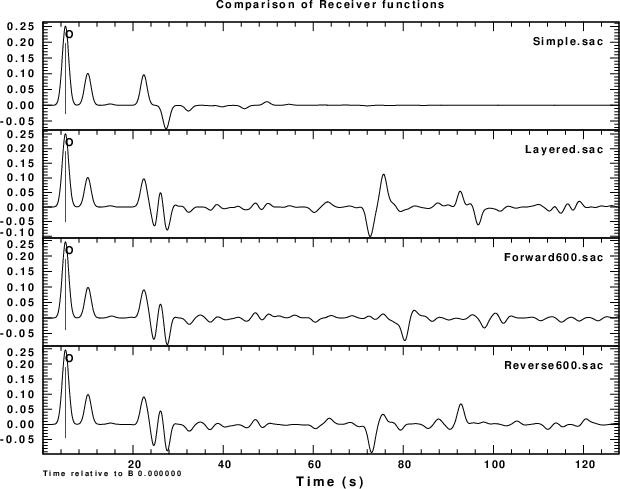This comparison shows that the difference at about 20s on the plot is due to the multiplayer structure. Comparing the arrival at about 72s of the Layered.sac and Reverse600.sac, shows an initial agreement. The differences may be due to the need for the model to be wider (?), or the need for more bounding rays in the Cerveny simulation. Recall that only 3 reverberations were permitted in the upper layers.

# Final thoughts

Additional model studies can be performed. These synthetics show structural features well because of the small number of layers and the sharp velocity contrasts. If there are gradients, then features will become less obvious.

# Notes

Charles Langston has a paper in the Bulletin, Seismological Society of America in 1977 on receiver functions in the Pacific Northwest of the U.S. This may be a good early reference.

# References

Červený, V., I. A. Molotkov, and I. Pšenčík, 1977, Ray Method in Seismology. Praha: Universita Karlova.

Červený, V. (1985). Seismic Ray Theory, DOI:  10.1007/0-387-30752-4_134

Červený, V. (2010). Seismic Ray Theory, Cambridge University Press, https://doi.org/10.1017/CBO9780511529399

Červený, V. and I. Pšenčík (2011). Seismic ray theory, http://sw3d.cz/papers.bin/r20vc1.pdf, 24pp.

Herrmann, R. B. (2013) Computer programs in seismology: An evolving tool for instruction and research, Seism. Res. Lettr. 84, 1081-1088, doi:10.1785/0220110096

Langston, C. A. (1977). The effect of planar dipping structure on source and receiver responses for a constant ray parameter, Bull. Seism. Soc. Am. 67, 1029-1050,  https://doi.org/10.1785/BSSA0670041029